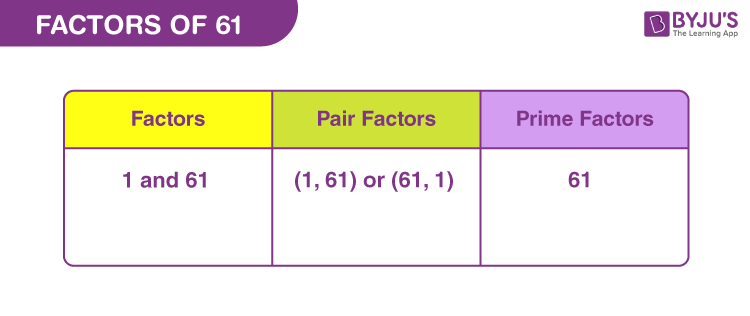# Factors of 61

Factors of 61 are the natural numbers that can divide the original number, uniformly. Thus, factors divide the original number into equal parts. 61 is a prime number, therefore, it is divisible by 1 and the number itself. 1 and 61 are also called the divisors of 61.

Alternatively, we can say, if the product of two natural numbers is equal to 61, then they are the factors of 61.## How to Find the Factors of 61?

61 divided by its factors, produces a whole number and the remainder is zero. Since, 61 itself is a prime number, therefore it is very easy to determine the factors of 61.

 61 ÷ 1 = 61 [Remainder = 0] 61 ÷ 61 = 1 [Remainder = 0]

Therefore, there are only two factors of 61. They are 1 and 61.

But if we divide 61 by any other number, then the result will not be a whole number but a fraction. Let us try some numbers.

61 ÷ 2 = 30.5

61 ÷ 5 = 12.2

61 ÷ 11 = 5.545454..

Thus, we can see, we are not able to find any other factors here.

## Pair Factors of 61

Pair factors are determined when the product of two numbers results in the original number.

• 1 × 61 = 61
• 61 × 1 = 61

Therefore, the pair factors of 61 are (1, 61) or (61, 1).

If we consider the negative factors in pairs to get 61, then;

• -1 × -61 = 61
• -61 × -1 = 61

Therefore, the negative pair factors are (-1, -61) or (-61, -1).

## Prime Factorisation of 61

Prime factorisation of 61 will generate the prime numbers that are the divisors of the original number. 61 is divisible by itself and no other prime factors.

61 ÷ 61 = 1

Therefore, the prime factor of 61 is 61 itself.

 Prime factorisation of 61 = 61 Exponential form = 611

## Solved Examples

Q.1: What is the sum of all the factors of 61?

Solution: The factors of 61 are 1 and 61.

Sum = 1 + 61 = 62

Therefore, the required sum is 62

Q.2: What are the common factors of 61 and 73?

Solution: We have to list the factors of 61 and 73.

61 → 1, 61

73 → 1, 73

Therefore, the common factor of 61 and 73 is 1.

Q.3: What are the greatest common factors of 61 and 65?

Solution: The factors of respective numbers are:

61 → 1, 61

65 → 1, 5, 13, 65

Therefore, GCF of 61 and 65 is 1.

### Practice Questions

1. Find the GCF of 61 and 51.
2. What are the common factors of 61 and 91.
3. What is the similarity between 61 and 97?
4. What are the common factors of 61, 82, 24, 81 and 70?

## Frequently Asked Questions on Factors of 61

### What are the factors of 61?

The factors of 61 are 1 and 61. Hence, there are two factors.

### Is 61 a prime number?

Yes, 61 is a prime number.

### What are the multiples of 61?

First ten multiples of 61 are 61, 122, 183, 244, 305, 366, 427, 488, 549 and 610.

### Why is 61 a prime number?

61 is a prime number because it has only two factors, 1 and the number itself.

### Is 61 a perfect square?

No, 61 is not a perfect square because the square root of 61 is a fraction. Thus, the square of no natural number is equal to 61.